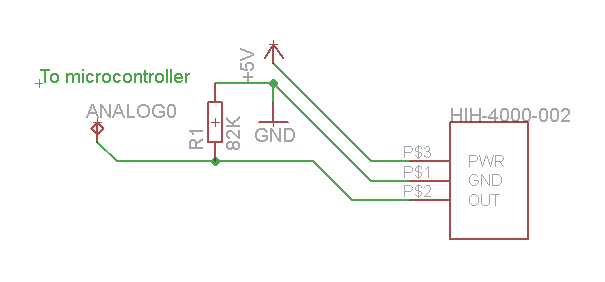## Humidity sensor HIH-4000 series with LCD-screen and Arduino

Here’s source code and information about my humidity sensor project. The LCD screen is connected following this datasheet from arduino examples. I might add photos of my setup later.And this is how I connected the humidity sensor:References:

Honeywell HIH-4000 datasheet

Arduino LCD Hello World example

Source code:

```// include the library code: #include <LiquidCrystal.h>   // initialize the library with the numbers of the interface pins LiquidCrystal lcd(12, 11, 5, 4, 3, 2); int sensorPin = 0; int sensorValue = 0; // variable to store the value coming from the sensor float voltage = 0; float RH = 0;   void setup() { // set up the LCD's number of rows and columns: lcd.begin(16, 1); }   void loop() { // read the value from the sensor: sensorValue = analogRead(sensorPin); // (1 = 0.0049V) 0-1023 range voltage = sensorValue * 0.0049; // Relative humidity(RH) (These are the values for my sensor, yours may differ a bit): // 0% = about 163 // 100% = about 795 // With roughly linear response. // 795 - 163 = 632 (points in the sensor's range) // 6.32 points = 1% RH RH = (sensorValue - 163) / 6.32; if (RH < -5) { lcd.setCursor(0,0); lcd.clear(); lcd.print("Check sensor!"); } else { lcd.clear(); lcd.setCursor(0, 0); lcd.print(sensorValue); //Analog input value in range of 0-1023 lcd.setCursor(5, 0); lcd.print(voltage); //Voltage calculated from the previous value, see comment above lcd.setCursor(10, 0); lcd.print(RH); //Relative humidity in percent } delay(100); }```

Update 17.04.2010: For those who want temperature correction and supply voltage correction (for use with for example DS2438), here’s how to calculate that:
Relative humidity = ((Voltage A/D)/(VCC) – 0.16) * 161.29 / 1.0546 – (0.00216 * (Temperature in C))